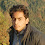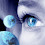## Thursday, October 13, 2011

### Generate random number in C++

Random number is generated by using srand(time(NULL)) and rand() function.For time we have to include time.h and for srand() and rand function we have to include stdlib.h.Here is the simple program which generate 10 random number.
`#include<iostream>`
`#include<time.h>`
`#include<stdlib.h>`
`#define MAX_NUM 10`
`using namespace std;`
`void randnum()`
`{`
`int random;`
`srand(time(NULL));`
`for(int i=0;i<10;i++)`
`{`
`random=rand()%MAX_NUM;`
`cout<<random<<endl;`
`}`
`}`
`int main()`
`{`
`cout<<"the ten random number is "<<endl;`
`randnum();`
`return 0;`
`}`

If you want to generate more or less then 10 random number then change the desire value of constant variable MAX_NUM.

1.Very good post on random number generation...but i think both srand() and rand() are declared inside stdlib.h...and also in c++ , i think the newer version has no time.h and stdlib.h . it has cstdlib and ctime..

2.ya you are right.I use codeblocks 10.05 where both run successfully and I love c rather than c++ so I forgot to write newer c++ style.

3.4.declaration error is shown in "using namespace std;" line :(

5.Really great!